# Class 9 RD Sharma Solutions – Chapter 10 Congruent Triangles- Exercise 10.1

• Last Updated : 03 Mar, 2021

### Question 1. In the figure, the sides BA and CA have been produced such that: BA = AD and CA = AE. Prove that segment DE || BC.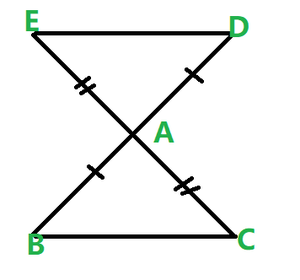Solution:

Given that:

BA = AD and CA = AE

Prove that DE || BC

So, here we consider triangle BAC and DAE,

CA= AE          -(given)

And ∠BAC = ∠DAE           -(vertically opposite angles)

Hence, by the SAS congruence criterion, we have

Δ BAC ≃ ΔDAE

So, we can say that:

∠DEA = ∠BCA, ∠EDA = ∠CBA          -(Corresponding parts of congruent triangles are equal)

Now, lines DE and BC are intersected by a transversal DB such that ∠DEA = ∠BCA.

Hence, proved that DE || BC.

### Question 2. In a Δ QPR, if PQ = QR and L, M and N are the mid-points of the sides PQ, QR, and RP respectively. Prove that LN = MN.

Solution: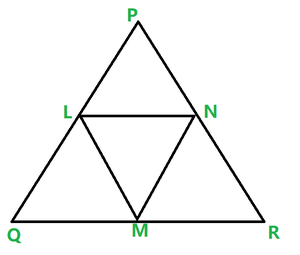Given that,

In Δ QPR, PQ = QR

Also, L, M, N are midpoints of the sides PQ, QP, and RP.

And we are given to prove that LN = MN.

In Δ QPR, PQ = QR, and L, M, N are midpoints

Hence, Δ QPR is an isosceles triangle.

PQ = QR

∠ QPR = ∠ QRP          -(1)

And also, L and M are midpoints of PQ and QR.

Hence, we can say that:

PQ = QR

PL = LQ = QM = MR = PQ/2 = QR/2

Now, we have Δ LPN and Δ MRN,

LP = MR

∠LPN = ∠MRN           -(From above)

∠QPR and ∠LPN are the same.

And also ∠QRP and ∠MRN are the same.

PN = NR          -(N is the midpoint of PR)

So, by SAS congruence criterion, we can say that ΔLPN = ΔMRN

By corresponding parts of congruent triangles are equal we can say that LN = MN.

### Question 3. In the figure given, PQRS is a square and SRT is an equilateral triangle. Prove that (i) PT = QT (ii) ∠TQR = 15°

Solution: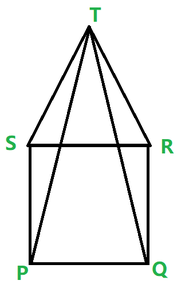We are given that PQRS is a square and SRT is an equilateral triangle.

And we have to prove that

(i) PT = QT

PQRS is a square and SRT is an equilateral triangle.

Now we see that PQRS is a square

PQ = QR = RS = SP        -(1)

And ∠SPQ = ∠PQR = ∠QRS = ∠RSP = 90° = right angle

And also, SRT is an equilateral triangle.

SR = RT = TS          -(2)

And ∠TSR = ∠SRT = ∠RTS = 60°

From equation(1) and (2) we can say that,

PQ = QR = SP = SR = RT = TS         -(3)

And also for angles we have,

∠TSP = ∠TSR + ∠RSP = 60° + 90° + 150°

∠TRQ = ∠TRS + ∠SRQ = 60° + 90° + 150°

∠TSR = ∠TRQ = 150°          -(4)

SP = RQ           -(From eq (3))

Hence, by SAS congruence criterion we can say that ΔTSP = ΔTRQ i.e both the triangles are congruent.

PT = QT          (Corresponding parts of congruent triangles are equal)

(ii) ∠TQR = 15°

Let’s see ΔTQR.

QR = TR

And also it is given that ΔTQR is an isosceles triangle.

∠QTR = ∠TQR         -(Angles opposite to equal sides)

As we know that the sum of angles in a triangle is equal to 180∘

∠QTR + ∠TQR + ∠TRQ = 180°

2∠TQR + 150° = 180°

2∠TQR = 180° – 150°

2∠TQR = 30°

Hence, ∠TQR = 15°

Hence, proved

### Question 4. Prove that the medians of an equilateral triangle are equal.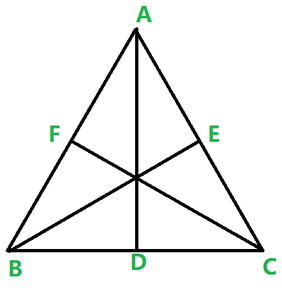Solution:

Prove that the medians of an equilateral triangle are equal.

So, let D, E, F are midpoints of BC, CA, and AB. AD, BE and CF are medians of ABC.

Then, AD, BE and CF are medians of ABC.

Now, we can say that

D Is the midpoint of BC

So now we can say that,

BD = DC = BC/2

CE = EA = AC/2

AF = FB = AB/2

Since, it is already given that ΔABC is an equilateral triangle

Therefore

AB = BC = CA

Hence, we can imply that,

BD = DC = CE = EA = AF = FB = BC/2 = AC/2 = AB/2

Also, we can say that ∠ABC = ∠BCA = ∠CAB = 60°        -(Angles of equilateral triangle)

Now, consider ΔABD and ΔBCE AB = BC

Here we find that BD = CE

Now, in ΔTSR and ΔTRQ

TS = TR

∠ABD = ∠BCE

So, from SAS congruence criterion, we can conclude that ΔABD = ΔBCE

Now, consider ΔBCE and ΔCAF, BC = CA

∠BCE = ∠CAF       (From above)

CE = AF

So, from SAS congruence criterion, we have, ΔBCE = ΔCAF

BE = CF [from above]

Hence proved

### Question 5. In ΔABC, if ∠A = 120° and AB = AC. Find ∠B and ∠C.

Solution:

In the triangle ABC

Given that ∠A = 120° and AB = AC

According to the question the triangle is isosceles

hence the angles opposite to equal sides are equal

∠B = ∠C

We also know that sum of angles in a triangle is equal to 180°

∠A + ∠B + ∠C = 180°

2∠B = 180° – 120°

∠B = ∠C = 30°

### Question 6. In ΔABC, if AB = AC and ∠B = 70°. Find ∠A.

Solution:

In the triangle ABC

Given that ∠B = 70° and AB = AC

According to the question the triangle is isosceles

hence angles opposite to equal sides are equal.

∠B = ∠C

Hence, ∠C = 70°

We also know that sum of angles in a triangle is equal to 180°

∠A + ∠B + ∠C = 180°

∠A = 40°

Hence, ∠A = 40° and ∠C = 70°

### Question 7. The vertical angle of an isosceles triangle is 100°. Find its base angles.

Solution:

Consider an isosceles ΔABC such that AB = AC

Given that vertical angle, ∠A = 100°

To find the base angles

Since ΔABC is an isosceles triangle hence ∠B = ∠C

We know that sum of interior angles of a triangle = 180°

∠A + ∠B + ∠C = 180°

100° + ∠B +∠B = 180°

2∠B = 180° – 100°

∠B = 40°

∠B = ∠C = 40°

Hence, ∠B = 40° and ∠C = 40°

### Question 8. In ∆ABC AB = AC and ∠ACD = 105°. Find ∠BAC.

Solution:

Given: AB = AC and ∠ACD = 105°

Since, ∠BCD = 180° = Straight angle

∠BCA + ∠ ACD = 180°

∠BCA + 105° = 180°

Hence, ∠BCA = 75°

Also, ΔABC is an isosceles triangle

AB = AC

∠ABC = ∠ ACB = 75°.

Now the Sum of Interior angles of a triangle = 180°

∠ABC = ∠BCA + ∠CAB = 180°

75° + 75° + ∠CAB = 180°

∠BAC = 30°

### Question 9. Find the measure of each exterior angle of an equilateral triangle.

Solution:

We know that for an equilateral triangle

∠ABC = ∠BCA = CAB =180°/3 = 60°

Now,

Extend side BC to D, CA to E, and AB to F.

∠BCA + ∠ACD = 180°

60° + ∠ACD = 180°

∠ACD = 120°

Similarly, we can say that, ∠BAE = ∠FBC = 120°

So, the measure of each exterior angle of an equilateral triangle = 120°.

### Question 10. If the base of an isosceles triangle is produced on both sides, prove that the exterior angles so formed are equal to each other.

Solution:

ED is a straight line segment and B and C are the points on it.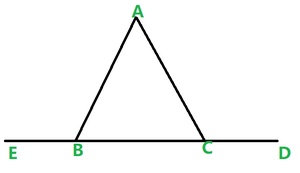∠EBC = ∠BCD          -(Both are equal to 180°)

∠EBA + ∠ABC = ∠ACB + ∠ACD

Since ∠ABC = ∠ACB

Hence, ∠EBA = ∠ACD

Hence, proved

### Question 11. In the given Figure AB = AC and DB = DC, find the ratio ∠ABD:∠ACD.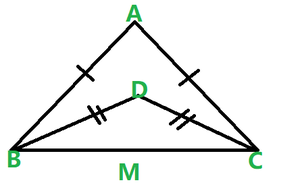Solution:

Given: AB = AC, DB = DC

∠ABD = ∠ACD

Hence, ΔABC and ΔDBC are isosceles triangles

∠ABC = ∠ACB and also ∠DBC = ∠DCB    (Angles opposite to equal sides are equal)

Now we have to find the ratio =∠ABD : ∠ACD

∠ABD = (∠ABC – ∠DBC)

∠ACD = (∠ACB – ∠DCB)

(∠ABC – ∠DBC):(∠ACB – ∠DCB)

(∠ABC – ∠DBC):(∠ABC – ∠DBC)

1:1

Hence, ∠ ABD:∠ ACD = 1:1

### Question 12. Determine the measure of each of the equal angles of a right-angled isosceles triangle.

Solution:

ABC is a right-angled triangle

∠A = 90° and AB = AC

Since,

AB = AC

∠C = ∠B

As we know that the sum of angles in a triangle = 180°

∠A + ∠B + ∠C = 180°

90° + ∠ B+ ∠ B = 180°

2∠B = 90°

∠B = 45°

∠B = 45°, ∠C = 45°

So, the measure of each of the equal angles of a right-angled Isosceles triangle = 45°

### Question 13. AB is a line segment. P and Q are points on opposite sides of AB such that each of them is equidistant from points A and B. Show that the line PQ is the perpendicular bisector of AB.

Solution: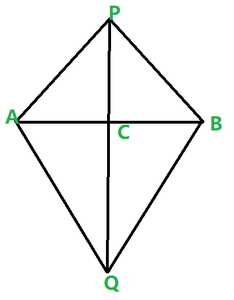Given:

AB is a line segment and P, Q are points on opposite sides of AB such that

AP = BP

AQ = BQ

Prove that PQ is the perpendicular bisector of AB.

Let ΔPAQ and ΔPBQ,

AP = BP

AQ = BQ

PQ – PQ

Δ PAQ ≃ Δ PBQ are congruent by SAS congruent condition.

Now, we can observe that APB and ABQ are isosceles triangles.

∠ PAB = ∠ ABQ

And also ∠ QAB = ∠ QBA

Now consider Δ PAC and Δ PBC

C is the point of intersection of AB and PQ

PA = PB

∠APC = ∠BPC

PC = PC

So, by SAS congruency of triangle ΔPAC ≅ ΔPBC.

AC = CB and ∠PCA = ∠PBC

And also, ACB is the line segment

∠ACP + ∠ BCP = 180°

∠ACP = ∠PCB

∠ACP = ∠PCB = 90°

We have AC = CB

C is the midpoint of AB

So, we can conclude that PC is the perpendicular bisector of AB and C is a point on the line PQ.

Therefore, PQ is the perpendicular bisector of AB.

My Personal Notes arrow_drop_up